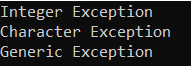# How to Throw and Catch Exception Between Functions in C++

## How to Throw and Catch Exceptions Between Functions in C++

In this article, I am going to discuss How to Throw and Catch Exception Between Functions in C++ with Examples. Please read our previous article where we discussed Exception Handling in C++ with Examples.

##### Throw and Catch Exception Between Functions in C++

Let us see How to Throw and Catch Exceptions Between Functions in C++. Already we have explained to you the format of the try and catch block in C++ in our previous article. Now let us understand one more important thing i.e. how to throw and catch exceptions between two functions. Please have a look at the below example code.

```#include <iostream>
using namespace std;
int main()
{
int a = 20, b = 0, c;
try
{
if (b == 0)
throw 1;
c = a / b;
cout << c;
}
catch (int e)
{
cout <<"Division by zero";
}
}
```

See, inside the try block, we are throwing the number and catching that number in the catch block. We can do the same thing using the if-else condition which is very simple as compared to try-catch. Then the question that should come to your mind is why are we using try and catch blocks? Now, let us understand the actual purpose of the try and catch blocks in C++. For a better understanding, please have a look at the below example.

```#include <iostream>
using namespace std;
int Division(int x, int y)
{
if (y == 0)
throw 1;
return x / y;
}

int main()
{
int a = 10, b = 0, c;
try
{
c = Division (a, b);
cout << c;
}
catch (int e)
{
cout << "Division by zero " << "error code " << e;
}
}
```

Here we have written two functions, which are main and Division. The Division function takes two integer type parameters, x, and y. In the main function, inside the try block, we have called the Division function with a and b parameters and stored the result in the c variable.

Now, in our example, the main function will not divide the two integers a and b, rather the Division function will divide a and b and return the result to the main function from where it is being called. Then, after storing the result of Division in the c variable, we will print the value of c. So why do we need the try and catch blocks?

When we call a function, that function will return the result. But what happens if a function is unable to return a result? Then what should the function do? Suppose a function cannot divide and continue, then what should the function do? The function should inform the calling function that there is some problem. We want the functions to communicate with one another. How? If a function is called by passing parameters, either it should return a result or it should inform the user or calling function about the error.

If the y parameter of the function Division is zero, then the function cannot perform the divide operation. So, to get information about the error, we have written some code in the Division function. Inside the division function, we have written an if condition that checks for if b == 0, then throw 1; otherwise, return x/y. Now the Division function will check for the error and inform the calling function about it. And if there is no error, then it will simply divide two integers and return the result.

If there is an exception in the Division function then it will throw that and that exception will be caught by the main function (as we defined catch block in the main function). Then no more lines will execute after getting the exception. After that, the catch block will be executed. If there is no exception then the try block will execute successfully and then the catch block will not execute. So, the try and catch block or throwing an exception is a communication between two functions.

For example, there are two functions, X and Y. X is called Y. X has given some parameters. Then what should X expect from Y? Result. Y will perform the operation and give X the result. Suppose Y is unable to solve this one because of some problem. Then what should Y do? If Y returns, then X will assume that it’s a result. So, Y will not return. Y will throw it. So, it means whenever X is giving some work to Y, then X should be ready for two things. Either Y gives the result. If everything is perfect or if there is any problem, Y will throw an exception. So, X should be ready to catch that exception. In this way, exception handling is more useful in between the functions; otherwise, the errors we can check just by using if and else conditions. So that’s it. This is an example of an exception. There are more things to learn about exceptions, and we will explain them to you in our upcoming articles.

##### Example to Understand How to Throw and Catch Exception Between Functions in C++
```#include <iostream>
using namespace std;

int Division(int a, int b) throw (int)
{
if (b == 0)
throw 1;
return a / b;
}

int main()
{
int x = 10, y = 2, z;
try
{
z = Division (x, y);
cout << z << endl;
}
catch (int e)
{
cout << "Division by zero " << e << endl;
}
cout << "Bye" << endl;
}
```
###### Output:

If x = 10 and y = 2,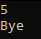If x = 10 and y = 0,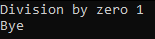##### Throwing Exceptions from C++ constructors

An exception should be thrown from a C++ constructor whenever an object cannot be properly constructed or initialized. Since there is no way to recover from failed object construction, an exception should be thrown in such cases. Since C++ constructors do not have a return type, it is not possible to use return codes. Therefore, the best practice is for constructors to throw an exception to signal failure. The throw statement can be used to throw a C++ exception and exit the constructor code. For a better understanding, please have a look at the below example.

```#include <iostream>
using namespace std;
class Rectangle
{
private:
int length;
public:
Rectangle(int l, int b)
{
if (l < 0 || b < 0)
{
throw 1;
}
else
{
length = l;
}
}
void Display()
{
cout << "Length: " << length << " Breadth: " << breadth;
}
};

int main()
{
try
{
Rectangle r1(10, -5);
r1.Display();
}
catch (int num)
{
cout << "Rectangle Object Creation Failed";
}
}
```

Now, if you pass any negative values for length and breadth while creating the Rectangle object, it will not create the object rather it will throw an exception as follows:

Rectangle Object Creation Failed

Now, if you pass any positive values for length and breadth while creating the Rectangle object, it will create the object and then it will invoke the Display method. Let us modify the main method as follows. Now we are passing 10 and 20 to the Rectangle constructor.

```int main()
{
try
{
Rectangle r1 (10,20);
r1.Display ();
}
catch (int num)
{
cout << "Rectangle Object Creation Failed";
}
}
```

Now, you will get the following output.

##### Points to Remember:
1. An exception in C++ is thrown by using the throw keyword from inside the try block. The throw keyword allows the programmer to define custom exceptions.
2. Exception handlers in C++ are declared with the catch keyword, which is placed immediately after the try block in C++. Multiple handlers (catch expressions) can be chained – each one with a different exception type. Only the handler whose argument type matches the exception type in the throw statement is executed.
3. C++ does not require a finally block to make sure resources are released if an exception occurs.
##### Using Multiple catch blocks in C++

The following example shows the use of multiple catch blocks to handle different types of exceptions. The following example code is self-explanatory. If the value of an integer in the array arr is less than 0, we are throwing a numeric value as an exception and if the value is greater than 0, then we are throwing a character value as an exception. And we have two different catch blocks to catch those exceptions.

```#include <iostream>
#include<conio.h>
using namespace std;

int main()
{
int arr = { -1, 2 };
for (int i = 0; i < 2; i++)
{
int num = arr[i];
try
{
if (num > 0)
// throwing numeric value as exception
throw 1;
else
// throwing a character/string as exception
throw 'a';
}
catch (int ex)	// to catch numeric exceptions
{
cout << "Integer Exception" << endl;
}
catch (char ex)	// to catch character/string exceptions
{
cout << "Character Exception" << endl;
}
}
return 0;
}
```
###### Output: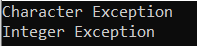##### Generic Catch Block in C++

The following example contains a generic catch block to catch any uncaught errors/exceptions. catch(…) block takes care of all type of exceptions.

```#include <iostream>
#include<conio.h>
using namespace std;

int main()
{
int arr = { -1, 2 };
for (int i = 0; i < 2; i++)
{
int num = arr[i];
try
{
if (num > 0)
// throwing numeric value as exception
throw 1;
else
// throwing a character/string as exception
throw 'a';
}
//Generic catch block
catch (...)	// to catch anytime of exceptions
{
cout << "Generic Exception" << endl;
}
}
return 0;
}
```
###### Output: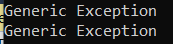In the below example, both the exceptions are being caught by a single catch block. We can even have separate catch blocks to handle integer and character exceptions along with the generalized catch block as shown in the below example.

```#include <iostream>
#include<conio.h>
using namespace std;

int main()
{
int arr = { -1, 2, 5 };
for (int i = 0; i < 3; i++)
{
int num = arr[i];
try
{
if (num == -1)
// throwing numeric value as exception
throw 1;
else if (num == 2)
// throwing a character/string as exception
throw 'a';
else
throw "Generic";
}
catch (int ex)	// to catch numeric exceptions
{
cout << "Integer Exception" << endl;
}
catch (char ex)	// to catch character/string exceptions
{
cout << "Character Exception" << endl;
}
//Generic catch block
catch (...)	// to catch anytime of exceptions
{
cout << "Generic Exception" << endl;
}
}
return 0;
}
```
###### Output: